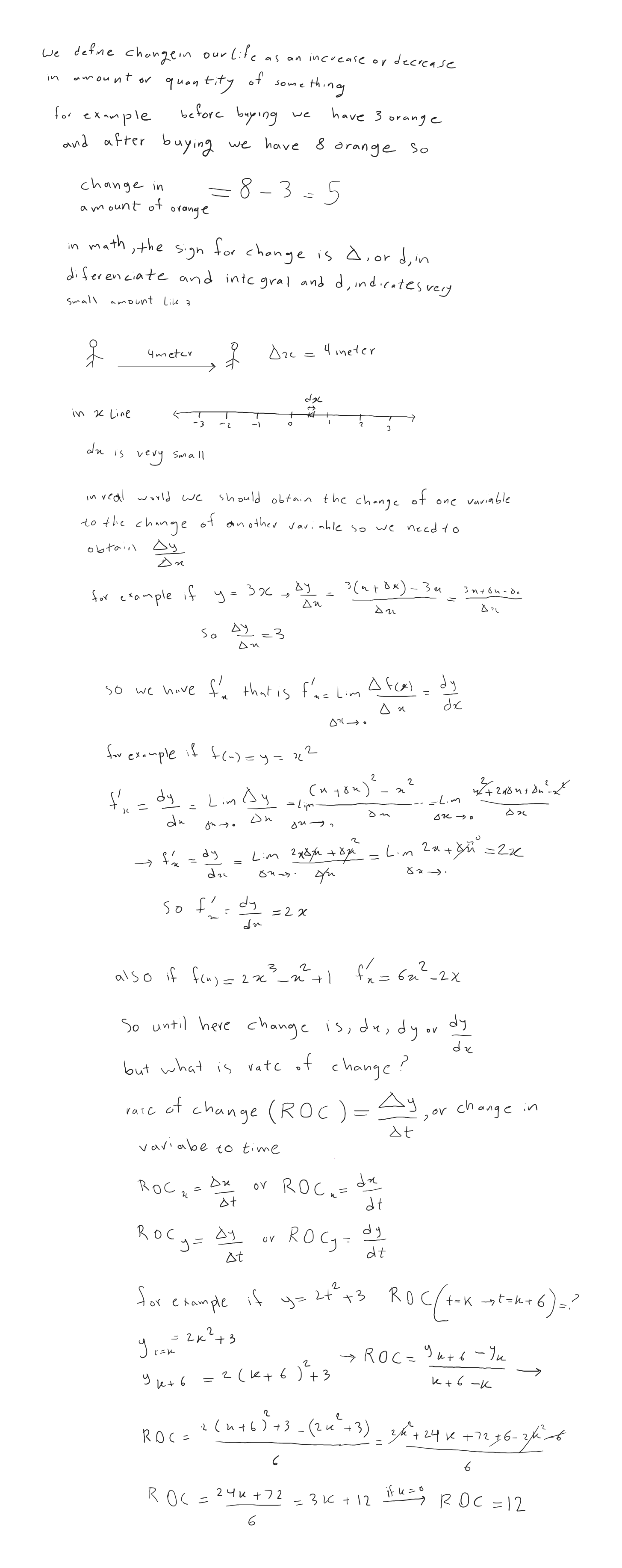SherpaMaths

>

GCSE

Rates of Change

Question

# How do you find the rate of change?

1 year ago

·

128 Replies

·

4492 views

V

Vickie Shanahan

M
Milton Tom

Frays of change is how much x. Ganges w time or another variable . So this can be done by working out the gradient of a graph or differentiating If it is an expression

Y
Yunho Kwon

Linear functions have a constant rate of change which is represented as the gradient of the graph.

It is simply the change of y-values with respect to x-values i.e. (y1-y2) / (x1-x2) where (x1,y1), (x2,y2) are the coordinates

L
Leon Cragg

the change in y-values by the change in x-values.

A
Amber Cummins

There are a couple of ways to find this. If your data is displayed in graph format, you can find the rate of change by dividing the change in y-values by the change in x-values. If you are talking about the instantaneous rate of change (this is called the derivative of a function) then we need to look further at the function itself.

A
Aamir Zaib

you take derivative with respect to time

S
Svm tujtuj

To find out the rate at which a value has changed we find the difference between the 2 values first. We then find the difference as a percentage of the original value this is done by dividing the difference by the original value and then multiplying it by 100. This can be demonstrated by: ((New Value -Original Value) / Original Value) x 100.

Pia Verified Sherpa Tutor ✓

Science & Maths tutor - A-Level, GCSE & KS3 Struggling/Higher Attainers

1 reviews

find the change in y over change in x using triangle on give graph

I'm available for 1:1 private online tuition!

I
Iliirjan

The rate of change in measured the change in Y over the change in X

Emanuel Verified Sherpa Tutor ✓

Experienced and enthusiastic Maths Tutor and Maths teacher of GCSE and

You would have to differentiate

I'm available for 1:1 private online tuition!

S
Sonya Pervez

The rate of change (ROC) is how much the value of something changes over a period of time and it is given in percentage terms.

To find this you first calculate the change in "Y" values (or the change in an outcome variable) divided by the change in "X" values (or the change in input variables).

So if my tutoring time increases from 3 hours in week 1 to 16 hours in week 2 and my income increases from £45 per week to £240 per week, Y values are my income and X values are the work hours I put in. r

To calculate the ROC I would do the following.

(240 - 45) / (16 - 3) = 195 / 13 = 15% rate of change in my earnings between week 1 and week 2.

DR M Verified Sherpa Tutor ✓

An experienced tutor for science subjects and competitive exams

If you take the change in Y values and divide that with the change in X-values the result will be a rate of change,

I'm available for 1:1 private online tuition!

Vashisht K Verified Sherpa Tutor ✓

4 years teaching GCSE. Engineering Student at King's College London

2 reviews

In a graph of a value vs time, it would be the gradient. Otherwise simply divide the difference in any particular value by the time taken.

I'm available for 1:1 private online tuition!

P
Paul Hunt

This is easier to work out from a graph, the rate of change is the gradient of the line - the change in y divided by the change in x. However, you might not have time in exam conditions to draw a graph when it's not asked of you, so I would suggest picking two points of the data - ignoring outliers if there are any. Calculate the difference between them by taking them away from each other, then divide that by the difference in time between them. This will give you the rate of change.

F
Farooq

The rate of change (ROC) is the speed at which a variable changes over a specific period of timeU
Unai Llona Carbajo

Derivatives are the best tool, but not for everyone... Considering two variables (distance and time, for example), you can also take the first and last value of both in a certain process, subtract the ones from the same variable (e.g. last and first distances of the bus from your position) and divide the subtractions of different variables. By doing this with position/distance and time, the rate of change you get is the speed of the object you're analyzing!

Think you can help?## Need a GCSE Maths tutor?

Get started with a free online introductions with an experienced and qualified online tutor on Sherpa.

Find a GCSE Maths Tutor